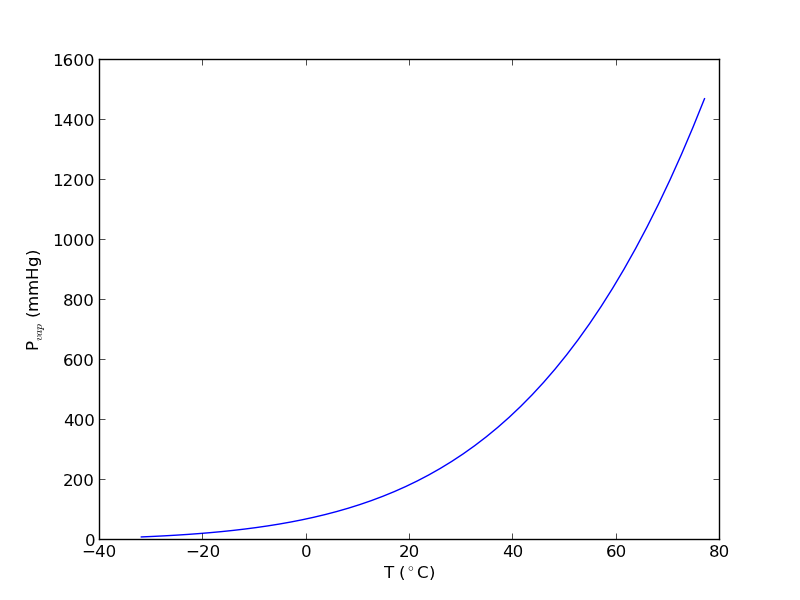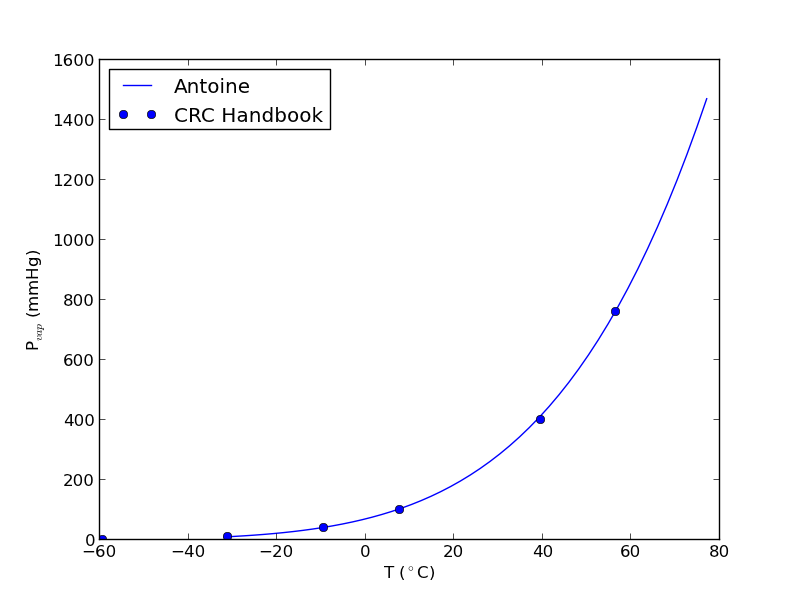## Reading in delimited text files

| categories: io | tags: | View Comments

sometimes you will get data in a delimited text file format, .e.g. separated by commas or tabs. Matlab can read these in easily. Suppose we have a file containing this data:

1   3
3   4
5   6
4   8


It is easy to read this directly into variables like this:

import numpy as np

x,y = np.loadtxt('data/testdata.txt', unpack=True)

print x,y

[ 1.  3.  5.  4.] [ 3.  4.  6.  8.]


Copyright (C) 2013 by John Kitchin. See the License for information about copying.

org-mode source

## Reading parameter database text files in python

| categories: io | tags: thermodynamics | View Comments

The datafile at http://terpconnect.umd.edu/~nsw/ench250/antoine.dat (dead link) contains data that can be used to estimate the vapor pressure of about 700 pure compounds using the Antoine equation

The data file has the following contents:

Antoine Coefficients
log(P) = A-B/(T+C) where P is in mmHg and T is in Celsius
Source of data: Yaws and Yang (Yaws, C.  L.  and Yang, H.  C.,
"To estimate vapor pressure easily. antoine coefficients relate vapor pressure to temperature for almost 700 major organic compounds", Hydrocarbon Processing, 68(10), p65-68, 1989.

ID  formula  compound name                  A       B       C     Tmin Tmax ??    ?
-----------------------------------------------------------------------------------
1 CCL4     carbon-tetrachloride        6.89410 1219.580 227.170  -20  101 Y2    0
2 CCL3F    trichlorofluoromethane      6.88430 1043.010 236.860  -33   27 Y2    0
3 CCL2F2   dichlorodifluoromethane     6.68619  782.072 235.377 -119  -30 Y6    0


To use this data, you find the line that has the compound you want, and read off the data. You could do that manually for each component you want but that is tedious, and error prone. Today we will see how to retrieve the file, then read the data into python to create a database we can use to store and retrieve the data.

We will use the data to find the temperature at which the vapor pressure of acetone is 400 mmHg.

We use numpy.loadtxt to read the file, and tell the function the format of each column. This creates a special kind of record array which we can access data by field name.

import numpy as np
import matplotlib.pyplot as plt

dtype=[('id', np.int),
('formula', 'S8'),
('name', 'S28'),
('A', np.float),
('B', np.float),
('C', np.float),
('Tmin', np.float),
('Tmax', np.float),
('??', 'S4'),
('?', 'S4')],
skiprows=7)

names = data['name']

acetone, = data[names == 'acetone']

# for readability we unpack the array into variables
id, formula, name, A, B, C, Tmin, Tmax, u1, u2 = acetone

T = np.linspace(Tmin, Tmax)
P = 10**(A - B / ( T + C))
plt.plot(T, P)
plt.xlabel('T ($^\circ$C)')
plt.ylabel('P$_{vap}$ (mmHg)')

# Find T at which Pvap = 400 mmHg
# from our graph we might guess T ~ 40 ^{\circ}C

def objective(T):
return 400 - 10**(A - B / (T + C))

from scipy.optimize import fsolve
Tsol, = fsolve(objective, 40)
print Tsol
print 'The vapor pressure is 400 mmHg at T = {0:1.1f} degC'.format(Tsol)

#Plot CRC data http://en.wikipedia.org/wiki/Acetone_%28data_page%29#Vapor_pressure_of_liquid
# We only include the data for the range where the Antoine fit is valid.

Tcrc  = [-59.4,         -31.1,  -9.4,   7.7,    39.5,   56.5]
Pcrc = [        1,      10,     40,     100,    400,    760]

plt.plot(Tcrc, Pcrc, 'bo')
plt.legend(['Antoine','CRC Handbook'], loc='best')
plt.savefig('images/antoine-2.png')

38.6138198197
The vapor pressure is 400 mmHg at T = 38.6 degCThis result is close to the value reported here (39.5 degC), from the CRC Handbook. The difference is probably that the value reported in the CRC is an actual experimental number.Copyright (C) 2013 by John Kitchin. See the License for information about copying.

org-mode source